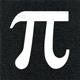Math Is Fun Forum

Discussion about math, puzzles, games and fun.   Useful symbols: ÷ × ½ √ ∞ ≠ ≤ ≥ ≈ ⇒ ± ∈ Δ θ ∴ ∑ ∫  π  -¹ ² ³ °

You are not logged in.

#1 2018-10-10 00:20:58

Βεν Γ. Κυθισ
MemberRegistered: 2018-10-09
Posts: 21

Repeated cosine converges!

So if you have f_0 = cos(n) and then f_1 = cos(f_0) and so on to get f_x = cos(f_(x-1)), and then let n be any real number, f_x converges to approximately

If you know anything that might relate to this new irrational number please respond to this post!

Edit:

I found out what this is, so don't clog the post with what you think it means, its meaning has already been revealed.

Last edited by Βεν Γ. Κυθισ (2018-10-10 10:11:05)

Offline

#2 2018-10-10 01:34:18

zetafunc
ModeratorRegistered: 2014-05-21
Posts: 2,376
Website

Re: Repeated cosine converges!

Hi Βεν,

Nice contribution! Yes, the repeated iteration of the cosine function converges: actually, it converges to the fixed point of the cosine function, i.e. the solution to
. (I think the two different answers come from using degrees versus radians rather than real vs imaginary.) There are ways of calculating this in terms of the Lambert W function or some nice infinite sums of Bessel functions I think.

You can prove that a solution to the above equation exists via Brouwer's fixed point theorem (and probably the contraction mapping theorem too).

Offline

#3 2018-10-10 09:58:45

Βεν Γ. Κυθισ
MemberRegistered: 2018-10-09
Posts: 21

Re: Repeated cosine converges!

zetafunc wrote:

Hi Βεν,

Nice contribution! Yes, the repeated iteration of the cosine function converges: actually, it converges to the fixed point of the cosine function,

That makes so much sense! I also just tried

and it converged to its fixed point, 0. Tan has a fixed point but it is not attractive; sinh becomes a vertical line on 0 which means there is no attractive point, 0; cosh has no fixed point but it converges to ∞; 0 is tanh's attractive fixed point; cot has no attractive point due to it being tan with the x axis shifted; sec is related to tan because it shares half of its discontinuous points with tan suggesting it has no attractive point, and it does have no attractive point; csc is sec with the x axis shifted so it has no fixed point.

Last edited by Βεν Γ. Κυθισ (2018-10-10 11:41:26)

Offline